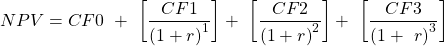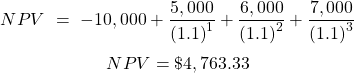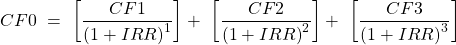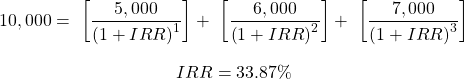Concept 2: Net Present Value (NPV) & Internal Rate of Return (IRR) | IFT World
101 Concepts for the Level I Exam

# Concept 2: Net Present Value (NPV) & Internal Rate of Return (IRR)The NPV of an investment is the present value of its cash inflows minus the present value of its cash outflows. The NPV of a project is calculated as:Consider a project which requires an initial investment of $10,000. It is expected to generate$5,000 in the first year, $6,000 in the second year and$7,000 in the third year. The cost of capital for this project is 10%. Calculate the NPV of this project.

Solution:Using the financial calculator: CF0 = ­-10,000; CF1 = 5,000; CF2 = 6,000; CF3 = 7,000; I = 10. CPT NPV = 4,763.33

The IRR is the discount rate the makes the NPV equal to zero. i.e. it equates the PV of the cash inflows to the PV of the cash outflows. The IRR of a project is calculated as:Consider a project which requires an initial investment of $10,000. It is expected to generate$5,000 in the first year, $6,000 in the second year and$7,000 in the third year. Calculate the IRR of this project.

Solution:Using the financial calculator: CF0 = -10,000; CF1 = 5,000; CF2 = 6,000; CF3 = 7,000; I = 10; CPT IRR = 33.87

LIVE WEEKEND CLASSES *For 2024 Exam candidates*
This is default text for notification bar